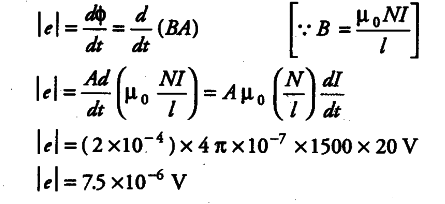# A long solenoid with 15 turns per cm has a small loop of area 2.0 cm

A long solenoid with 15 turns per cm has a small loop of area 2.0 \${{cm}^{2}}\$ placed inside, normal to the axis of solenoid. If the current carried by the solenoid changes steadily from 2 A to 4 A in 0.1 s, what is the induced voltage in the loop, while the current is changing?

Here, number of turns per unit length,
n= N/l = 15 turns/cm=1500 turns/m
A = 20 {{cm}^{2}} = 2 x \${{10}^{-4}}\$\${{m}^{2}} dI/dt = 4-2/0.1 or dI/dt = 20 A{{s}^{-1}}\$